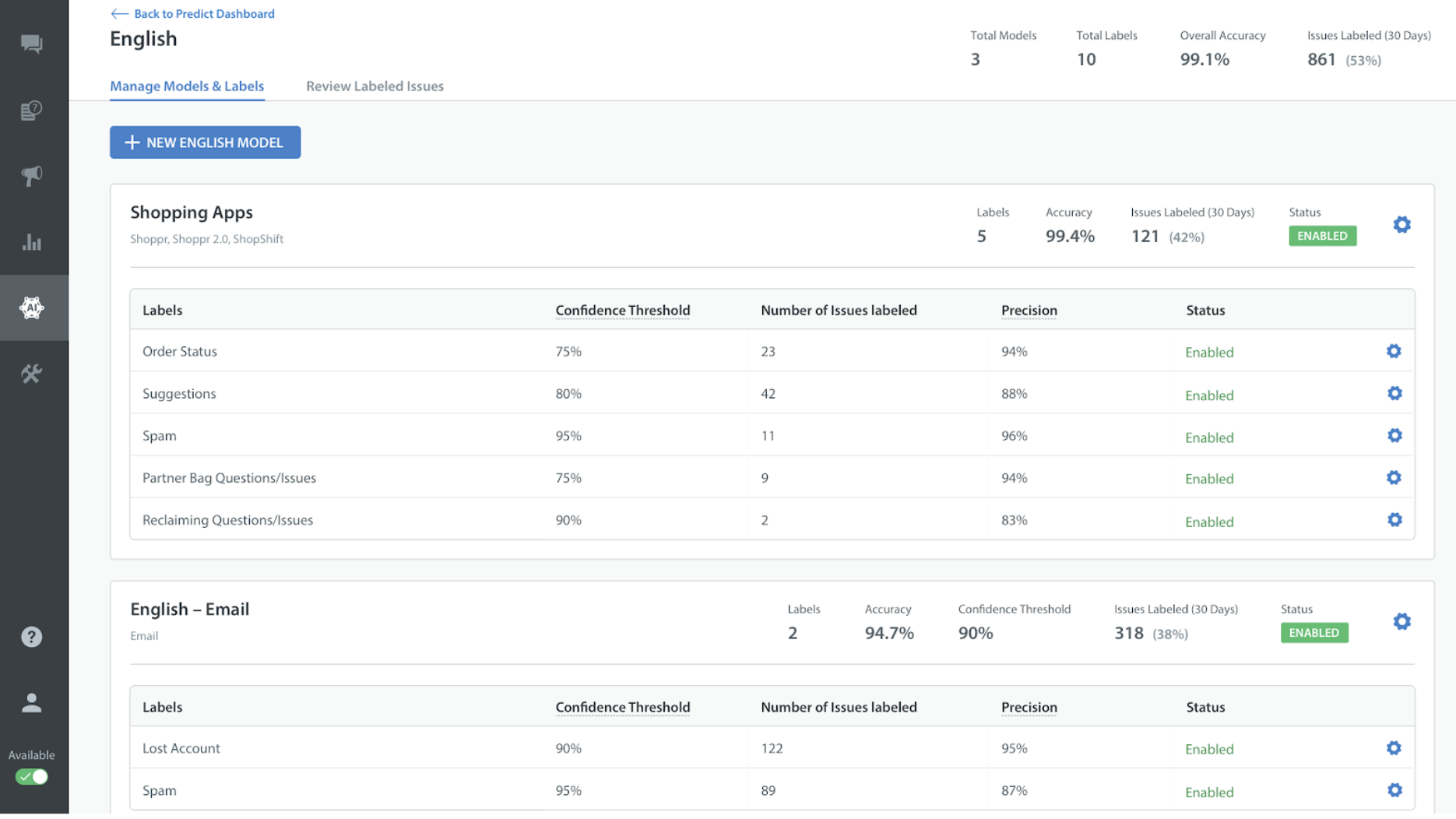Accuracy is calculated as the percentage of times that an Agent did not mark the predicted Label as wrong.

Accuracy reflects the Model’s performance. Your Accuracy calculation includes all Labels within the Model.

The formula for Accuracy is: (N-r) / (N)

N: Number of Issues for which any Label was predicted

r: Number of Issues for which any predicted Label was changed to another Label or marked as wrong

Precision is calculated as the percentage of times an Agent did not mark a specific Label as wrong when it was predicted. Precision is calculated per Label, so it can be different for each Label.

The formula for Precision is: (L-w) / L

L: Number of Issues for which a specific Label was predicted

w: Number of Issues for which a specific predicted Label was changed to another Label or marked as wrongFor example, let’s say you have an English Model with two Labels, ‘Orders’ and ‘Billing’. Of the 1000 Issues that were evaluated by this Model, 300 of those Issues were labeled as Orders, and 500 of those Issues were labeled as Billing.

Of the 300 Issues labeled as Orders, 60 of those Labels were corrected to Billing by your Agents, and 30 of those Labels were marked as wrong. In comparison, of the 500 Billing labels, 20 were corrected to Orders by your Agents, and 50 were marked as wrong.

The formula for Precision as applied to these Labels is as follows:

• Precision for Orders: (300 – 60 – 30) / (300) = 210 / 300 = 70%
• Precision for Billing: (500 – 20 – 50) / (500) = 430 / 500 = 86%

The formula for Accuracy for this Model is as follows:

For Issues with feedback:

• Orders: (60 + 30) = 90
• Billing: (20 + 50) = 70

Total Issues with feedback: 90 + 70 = 160

Total Issues labeled: 300 + 500 = 800

Thus, Accuracy for this Model is calculated as: (800 – 160) / (800) = 80%

We only calculate Precision and Accuracy once Issues are Resolved or Rejected. That way, these Labels can still be updated while the Issue is open, and your analytics will not reflect the new metrics until the Issue is closed.

The higher your Accuracy and Precision metrics are, the better. If these metrics are not meeting your expectations, you may wish to consider uploading more data or evaluating the data that is associated with the Labels for that Model. To learn more, see How do I prepare my data for the Predict Model?